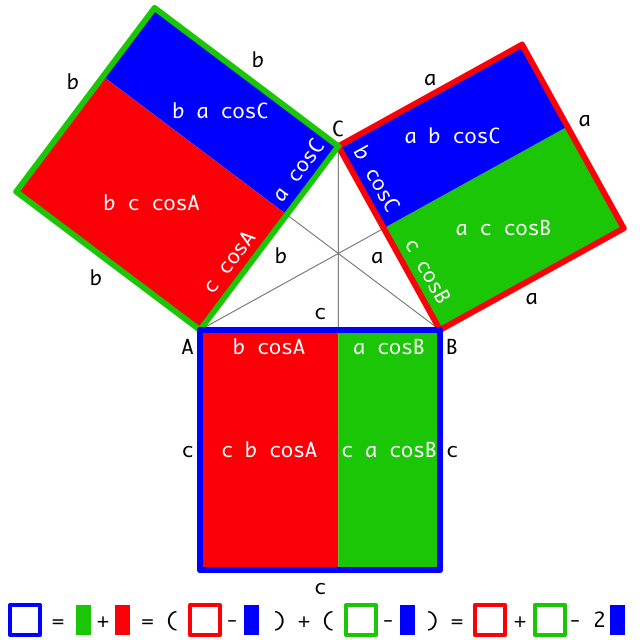# The Illustrated Law of Cosines

### Don McConnell August 25, 2010

What I have is a proof of the Law of Cosines that I believe to be original (and very cut-the-knot-ty, if I do say so myself):Drop three perpendiculars and let the definition of cosine give the lengths of the sub-divided segments. Then, observe that like-colored rectangles have the same area (computed in slightly different ways), and the result follows immediately.

When C is a right angle, the blue rectangles vanish and we have the Pythagorean Theorem via what amounts to Proof #5 on Cut-the-Knot's Pythagorean Theorem page. (Note that, as mentioned on CTK, the use of cosine here doesn't amount to an invalid "trigonometric proof".) It seems that Proof #65 may also be a relative.

The picture can be adjusted for obtuse C as well. (Proof left as an exercise for the reader.)

A final note ... Because the same-colored rectangles have the same area, they're "equidecomposable" (aka "scissors congruent"): it's possible to cut one into a finite number of polygonal pieces that reassemble to make the other. While there's at least one standard procedure for determining how to make the cuts, the resulting pieces aren't necessarily pretty. Some popular dissection proofs of the Pythagorean Theorem --such as Proof #36 on Cut-the-Knot-- demonstrate a specific, clear pattern for cutting up the figure's three squares, a pattern that applies to all right triangles. I have yet to find a similarly straightforward cutting pattern that would apply to all triangles and show that my same-colored rectangles "obviously" have the same area.

(Another exercise for the reader, perhaps? :)